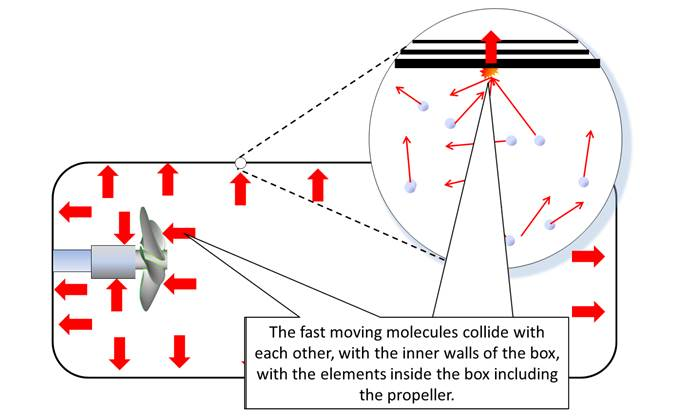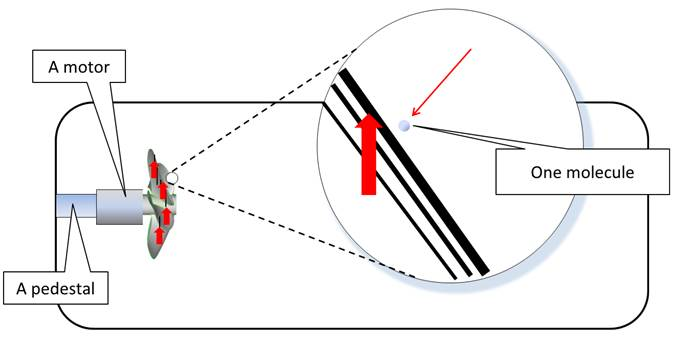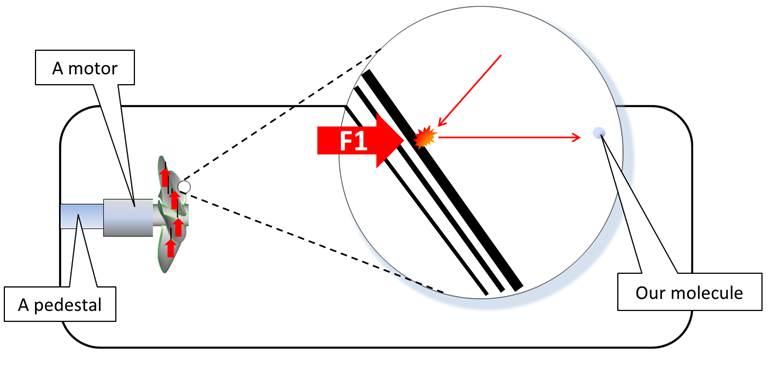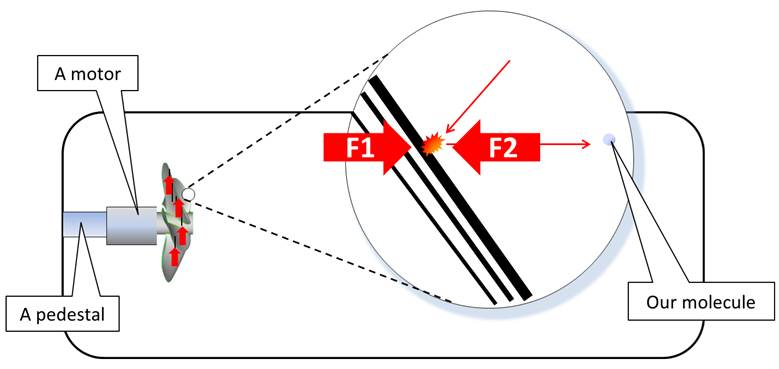WJETech (we push from the inside)

Tecnologías William Jhon Elliott Somerville E.I.R.L.

Rut: 76722619-5

So how does this thing work again?

Please see main page

THIS PAGE IS A WORK IN PROGRESS

Videos of simple demonstration model

NOTE: yes we have noticed that in the video the walls flex, but it is not sufficient to propel the box, yes there is a slight vibration of the motor but we do not believe it is sufficient to move the box, we have:

Turned the motor on without propeller (it just vibrates).

Replaces propeller with small flywheel, no motion.

Tried a propeller with reversed pitch angle (so motor must be reversed to blow in the backwards direction).

Used two counter rotating propellers.

Tried on Torsion pendulum, balanced horizontal pendulum, little boat and hanged from 2.2 meter altitude where when motor is turned on it swings 0.0781 degrees (a 3 mm displacement and stays there)Fig 1

So we have our airtight box and inside is a motorized propeller (details), when we turn the motor on, the box turns/moves. As basic physics clearly states that an object at rest cannot move UNLESS a force is applied to it, if no OUTSIDE force is applied (careful with a breeze) then the force is INSIDE the box.

But basic physics also states that a closed system (airtight box) cannot push itself from the inside, it MUST expel mass (like a rocket), and yet we can clearly see in video 1 that the airtight box IS pushing itself from the inside.

Physics cannot have it both ways, here we have a conflict between our basic physics (law of conservation of linear momentum) and what we observe, someone is mistaken.

Here is where we remember what Richard Feynman said.

Let us take a closer look inside the airtight box, first we must remember that although the box LOOKS empty to us, in reality there are 54.634.954.084.936.200.000.000.000 molecules (that’s 54 septillion ) very fast moving molecules colliding with each other in random manner (fig 2)Fig 2

The fast moving molecules collide with each other, with the inner walls of the box, with the elements inside the box including the propeller.

When the propeller is activated it collides with billions of air molecules with a strong force sending them in toward the rear of the box interacting with billions and billions of molecules before affecting the rear of the box

To simplify we will take a look at the idealized interactions of ONE molecule (Fig 3).Fig 3

When the propeller blade collides with the molecule it applies a strong force (F1) on the molecule, accelerating it towards the rear of the box (Fig 4).Fig 4

As for every action there is an equal and opposite reaction the propeller blade receives a force (F2) that pushes it (and the box) forward.Fig 5

As the molecule was accelerated its momentum increases (momentum = velocity x mass) and it is traveling with that momentum to the rear of the box (Fig. 6).Fig. 6

If the molecule is very lucky and it reaches the box’s rear wall without interacting with any of the 54 septillion molecules inside the box (very lucky indeed) it will collide with the rear inner wall with exactly the same force (F3) that the propeller received (F2), the forces pushing the box forward (F2) and the force pushing the inner rear end of the box (F3) are equal (Fig. 6)Fig. 6

If every molecule that collides with the propeller reaches the rear inner wall with its momentum unchanged, the box will not move (Fig. 7)Fig 7

But few molecules will get that lucky, most will collide with billions of molecules and their VECTOR DIRECTION will be randomized, many will not reach the rear inner wall with the same force.

Let’s take a closer look at two molecules colliding and how the collision affects their momentum.

Fig. 8 shows the first molecule 1 (M1) that is traveling towards the box’s rear inner wall, it has a mass (m1) and a velocity (v1)

Momentum is mass x velocity therefore its momentum is m1 x v1 = p1Fig. 8

Fig. 9 shows that molecule 1 is about to collide with incoming molecule 2 (M2) it has a mass (m2) and a velocity (v2)

Momentum is mass x velocity therefore its momentum is m2 x v2 = p2

If we add their momentum we get p1 + p2 = p (f)Fig. 9

If the two molecules collide there are some changes (Fig. 10)Fig. 10

 Molecule 1 (M1) before collision Molecule 1 (M1)  after collision Mass = m1 Mass = m1 (no change) Velocity = v1 Velocity may increase or decrease after the collision = v1(+ or -) Momentum = m1 x v1 = p1 Momentum = m1 x v1(+ or -) = p(a), the momentum of the individual molecule may change Molecule 2 (M2) before collision Molecule 2 (M2) after collision Mass = m2 Mass = m2 (no change) Velocity = v2 Velocity may increase or decrease after the collision = v2(+ or -) Momentum = m2 x v2 = p2 Momentum = m2 x v2(+ or -) = p(b), the momentum of the individual molecule may change Momentum of the SYSTEM (M1 + M2) before the collision Momentum of the SYSTEM (M1 + M2) after the collision p1 + p2 = p(s) p(a) + p(b) = p(s) Therefore:      p1 + p2 = p(a) + p(b) = p(s) The momentum of the SYSTEM (both molecules) DOES NOT CHANGE

Conclusion

The collision will not change the mass of the molecules

The collision may change the velocity of the molecules

The collision may change the momentum of individual molecules

The momentum of the system composed of both molecules does not change (in magnitude).

In order to describe momentum its magnitude is not sufficient, we also need its direction, and we describe its movement using a 3 axis graph (vector graph)Fig 11

Fig 11 illustrates the vector movement of molecule 1 (M1) it has a positive value in the X axis, 0 value in the Y and Z axis because it is moving straight forward.Fig 12

Fig 12 illustrates the vector movement of molecule 2 (M2) it has a positive value in the Z axis (going up), negative values in the X and Z axis, those values describe its vector direction.Fig 13

Fig 13 illustrates how the vectors of both molecules are changed, we have no way (that I know of) to predict the X, Y and Z values of each molecule, every collision will randomize their direction making it almost impossible that they reach the box’s rear end uninterrupted.

And that explains (I think) why not enough molecules collide with the rear inner wall of the box to totally cancel the forces excreted by molecules to the propeller and the box accelerates without outside interference